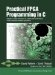# Section 7.5. Unrolling Loops

### 7.5. Unrolling Loops

As described in the previous section, the optimizer automatically parallelizes across expressions and statements within a basic block of C code. The optimizer does not, however, automatically parallelize operations across iterations of a loop. Loop unrolling is one technique that can be used to parallelize a loop by turning the loop into one basic block of straight-line code.

If the number of iterations of a loop is known at compile time, it is possible to unroll the loop to create dedicated logic for each iteration of the loop. Unrolling simply duplicates the body of the loop as many times as there are iterations in the loop.

For example, consider the following loop:

` for (i=0; i<10; i++) {     sum += A[i]; } `

Without unrolling, this loop will generate logic to perform each iteration in two cycles. The first cycle will read from memory A, and the second cycle will calculate the addition. One adder is generated that will be used ten times during execution of the loop. Now consider the same loop with unrolling:

` int i;    // Loop index must be type int ... for (i=0; i<10; i++) { #pragma CO UNROLL  sum += A[i]; } `

Unrolling simply duplicates the body of the loop for all values of i. In this example the result is equivalent to the following:

` sum += A; sum += A; sum += A; sum += A; sum += A; sum += A; sum += A; sum += A; sum += A; sum += A; `

In this case, ten adders are generated, and each one is used only once during the execution of the loop. Most of the time, as in this case, loop unrolling alone has no specific benefit. Only one value of A can be read in a given cycle, so this example loop still requires ten cycles to execute, and ten adders have been generated, which requires a lot of logic. However, if the "scalarize array variables" optimizer option is used together with loop unrolling, the elements of the array are replaced with scalar variables. The results would then be equivalent to the following:

` sum += A_0; sum += A_1; sum += A_2; sum += A_3; sum += A_4; sum += A_5; sum += A_6; sum += A_7; sum += A_8; sum += A_9; `

Instead of generating a memory for the array A, registers are generated for each of the ten elements of the array. All ten registers can be read simultaneously and thus this entire loop can be executed in a single cycle.

Note

The use of unrolling requires some care because a large amount of logic can be easily generated and the cycle delay can be greatly increased, with a corresponding decrease in maximum clock frequency. In the preceding example, ten adders will be generated, and because the result of each adder is used by the next adder, this cycle's delay is ten times the delay of a single adder's delay. You can keep this delay under control by placing a StageDelay pragma immediately preceding the loop.Practical FPGA Programming in C
ISBN: 0131543180
EAN: 2147483647
Year: 2005
Pages: 208

Similar book on Amazon